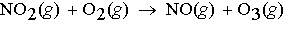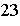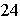Name:    Unit 7 Review

Multiple Choice
Identify the choice that best completes the statement or answers the question.

1.

Isotopes are atoms of the same element with different numbers of _____.
 a. neutrons. c. electrons. b. protons. d. all of these.

2.

Magnesium and calcium are alkaline earth metals, they form a ____ charge.
 a. 1- c. 2- b. 1+ d. 2+

3.

Which of the following equalities is correct?
 a. 0 K = 273�C c. -273 K = 0 �C b. 0 K = -273�C d. none of these are correct

4.

Which of the following is the correct electron configuration for carbon?
 a. 1s22p2 c. 1s22s12p3 b. 1s22s22p6 d. 1s22s22p2

5.

Which of the following uses correct symbols and coefficients to show the formation of water from gaseous hydrogen and oxygen?
 a. H2(g) + O(g) ® 2 H2O(l) c. 2H2(g) + O2(g) ® 2H2O(l) b. 2H2O(l) ® 2H2(g) + O2(g) d. 2H2(g) + O2(g) ® 2 H2O(aq)

6.

When a solid is produced in a chemical reaction that solid is called
 a. a reactant. c. a molecule. b. a precipitate. d. the mass of the product.

7.

In writing a chemical equation that produces hydrogen gas, the correct representation of hydrogen gas is
 a. ­. c. H2. b. ¯. d. 2H.

8.

What is the whole number that appears in front of a chemical formula in a chemical equation called?
 a. a coefficient c. a subscript b. a ratio d. a superscript

9.

According to the law of conservation of mass, the total mass of the reacting substances is
 a. always more than the total mass of the products. b. always less than the total mass of the products. c. sometimes more and sometimes less than the total mass of the products. d. always equal to the total mass of the products.

10.

A chemical equation is balanced when the
 a. products and reactants are the same chemicals. b. subscripts of the reactants equal the subscripts of the products. c. same number of each kind of atom appears in the reactants and in the products. d. coefficients of the reactants equal the coefficients of the products.

11.

In an equation, the symbol for a substance that is dissolved in water is followed by
 a. (1). c. (s). b. (aq). d. (g).

12.

A chemical formula written over the arrow in a chemical equation signifies
 a. an impurity. c. a catalyst for the reaction. b. the formation of a gas. d. a by-product.

13.

When the equation Fe3O4 + Al ® Al2O3 + Fe is correctly balanced, what is the coefficient of Fe?
 a. 3 c. 4 b. 6 d. 9

14.

Which coefficients correctly balance the formula equation
NH4NO2(s)® N2(g) + H2O(l)?
 a. 1, 1, 2 c. 1, 2, 2 b. 2, 2, 2 d. 2, 1, 1

15.

Which coefficients correctly balance the formula equation C3H7 + O2 ® CO+ H2O?
 a. 2, 19, 12, 7 c. 4, 19, 12, 14 b. 2, 10, 6, 5 d. 2, 38, 12, 7

16.

Which coefficients correctly balance the formula equation CaO + H2O ® Ca(OH)2?
 a. 2, 1, 2 c. 1, 1, 1 b. 1, 2, 1 d. 1, 2, 3

17.

In what kind of reaction do two or more substances combine to form a new compound?
 a. double-replacement reaction c. decomposition reaction b. synthesis reaction d. ionic reaction

18.

The equation CxHy+ O2 ® CO2 + H2O is the general equation for a
 a. synthesis reaction. c. single-replacement reaction. b. combustion reaction. d. decomposition reaction.

19.

The equation AX + BY ® AY + BX is the general equation for a
 a. single-replacement reaction. c. decomposition reaction. b. synthesis reaction. d. double-replacement reaction.

20.

The equation AX  ® A + X is the general equation for a(n)
 a. double-replacement reaction. c. synthesis reaction. b. decomposition reaction. d. combustion reaction.

21.

In what kind of reaction does a single compound produce two or more simpler substances?
 a. single-replacement reaction c. decomposition reaction b. ionic reaction d. synthesis reaction

22.

The equation A + BX ® AX + B is the general equation for a
 a. single-replacement reaction. c. double-replacement reaction. b. combustion reaction. d. decomposition reaction.

23.

The reaction represented by the equation 2Mg(s) + O2(g) ® 2MgO(s) is a
 a. single-replacement reaction. c. decomposition reaction. b. double-replacement reaction. d. synthesis reaction.

24.

The reaction represented by the equation Mg(s) + 2HCl(aq) ® H2(g) + MgCl2(aq) is a
 a. single-replacement reaction. c. composition reaction. b. decomposition reaction. d. double-replacement reaction.

25.

The reaction represented by the equation Pb(NO3)2(aq) + 2KI(aq) ® PbI2(s) + 2KNO3(aq) is a
 a. decomposition reaction. c. double-replacement reaction. b. synthesis reaction. d. combustion reaction.

26.

The reaction represented by the equation 2KClO3(s) ® 2KCl(s) + 3O2(g) is a(n)
 a. decomposition reaction. c. synthesis reaction. b. combustion reaction. d. ionic reaction.

27.

The coefficients in a chemical equation represent the
 a. number of atoms in each compound in a reaction. b. masses, in grams, of all reactants and products. c. number of valence electrons involved in the reaction. d. relative numbers of moles of reactants and products.

28.

A balanced chemical equation allows one to determine the
 a. energy released in the reaction. b. mole ratio of any two substances in the reaction. c. electron configuration of all elements in the reaction. d. mechanism involved in the reaction.

29.

The units of molar mass are
 a. amu/g. c. g/mol. b. mol/g. d. amu/mol.

30.

In the reaction represented by the equation N2 + 3H2 ® 2NH3, what is the mole ratio of nitrogen to ammonia?
 a. 1:1 c. 2:3 b. 1:2 d. 1:3

31.

For the reaction represented by the equation C + 2H2 ® CH4, how many moles of hydrogen are required to produce 10 mol of methane, CH4?
 a. 10 mol c. 20 mol b. 4 mol d. 2 mol

32.

For the reaction represented by the equation 2H2 + O2® 2H2O, how many liters of hydrogen are needed to completely react 80 grams of oxygen?
 a. 112 liters c. 3 liters b. 56 liters d. 224 liters

33.

For the reaction represented by the equation 2H2 + O2 ® 2H2O, how many grams of water are produced from 6.00 mol of hydrogen?
 a. 6.00 g c. 54.0 g b. 108 g d. 2.00 g

34.

For the reaction represented by the equation 2Na + Cl2 ® 2NaCl, how many grams of chlorine gas are required to react completely with 2.00 mol of sodium?
 a. 141.8 g c. 212.7 g b. 70.9 g d. 35.5 g

35.

For the reaction represented by the equation 2HNO3 + Mg(OH)2 ® Mg(NO3)2 + 2H2O, how many grams of magnesium nitrate are produced from 8.00 mol of nitric acid, HNO3, and an excess of Mg(OH)2?
 a. 445 g c. 148 g b. 818 g d. 593 g

36.

Ozone, O3, is produced by the reaction represented by the following equation:What mass of ozone will form from the reaction of 2.0 g of NO2 in a car's exhaust and excess oxygen?
 a. 1.1 g O3 c. 4.2 g O3 b. 2.1 g O3 d. 1.8 g O3

37.

For the reaction represented by the equation Cl2 + 2KBr ® 2KCl + Br2, how many grams of potassium chloride can be produced from 300. g each of chlorine and potassium bromide?
 a. 98.7 g c. 188 g b. 451 g d. 111 g

38.

For the reaction represented by the equation 2Na + 2H2O ® 2NaOH + H2, how many grams of hydrogen are produced if 120. g of sodium and 80. g of water are available?
 a. 4.5 g c. 45 g b. 80. g d. 200 g

39.

For the reaction represented by the equation SO3 + H2O ® H2SO4, how many grams of sulfuric acid can be produced from 200. g of sulfur trioxide and 100. g of water?
 a. 100 g c. 200 g b. 285 g d. 245 g

40.

Which reactant controls the amount of product formed in a chemical reaction?
 a. excess reactant c. limiting reactant b. composition reactant d. mole ratio

41.

A chemical reaction involving substances A and B stops when B is completely used. B is the
 a. primary product. c. excess reactant. b. limiting reactant. d. primary reactant.

42.

For the reaction represented by the equation C + 2H2 ® CH4, how many molecules of hydrogen are required to produce 1.5 mol of methane, CH4?
 a. 1 mole c. 9.0 x 10molecules b. 1.8 x 10molecules d. 3 moles

43.

The symbol D when found in a chemical reaction stands for
 a. reactants c. energy b. ice d. products

44.

In an exothermic reaction
 a. heat is given off as a product c. thermal energy is needed by reactants b. all of these are possible d. heat is required to start the reaction

45.

When the symbol D is found to the left of the yields sign (®), the reaction must be
 a. exothermic c. endothermic b. in water d. slow